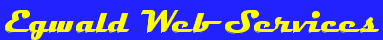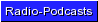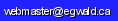Egwald Mathematics: Linear Algebra by Elmer G. Wiens Egwald's popular web pages are provided without cost to users. Please show your support by joining Egwald Web Services as a Facebook Fan:Follow Elmer Wiens on Twitter:The Mathematical Theory of Polynomials, Vectors, Matrices, Linear Equations, and Linear Differential Equations, with illustrated, interactive online examples The concepts and techniques of linear algebra are used extensively in the physical and social sciences to model phenomena of concern. 1. Investigate the different types of polynomials, their graphs, and their roots: polynomials and polynomial roots. 2. Investigate the properties of vectors, vector spaces, and linear transformations on the vectors web page. 3. Investigate matrices, matrix operations, orthogonal matrices, determinants, matrix inverses, eigenvalues and eigenvectors, generalized eigenvectors and the Jordan Normal Form, along with some important matrix decompositions on the matrices webpage. Matrix decompositions available include Gauss, Gram-Schmidt, Householder, Jacobi (Givens), and singular value decomposition. 4. Solve systems of linear equations, including underdetermined, overdetermined, and square matrix systems. Determine the particular and general solution of systems of linear equations with fewer rows than columns using the Echelon and Singular Value Decomposition algorithms. See the relationship between the image and kernel of a matrix. Use the "normal equations" and singular value decomposition methods to obtain the least squares solution to overdetermined systems of equations. Solve square matrix systems with the Gauss-Jordan, Gaussian, A = QR, and singular value decomposition methods. 5. Solve systems of linear differential equations, using the linear algebra from the preceeding pages. Includes a detailed section on the types of equilibria for a 2 by 2 system of linear differential equations.Copyright © Elmer G. Wiens:   Egwald Web ServicesAll Rights Reserved.    Inquiries